## Example Questions

### Example Question #41 : How To Find Synthesis Of Data In Biology

Consider the graph. A company is attempting to develop a new antibiotic to treat Staph infections. A petri dish of bacterial colonies are grown on LB plates with or without an antibiotic for 7 days. The percent survival of the bacterial colonies is plotted. Based on the data, a patient should take the antibiotic for a minimum of how many days?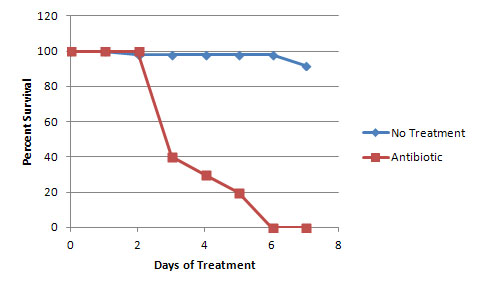5

2

6

3

6

Explanation:

After 5 days in culture, a low percentage of the colonies are still alive. However, after 6 days, 0% remain. Therefore, the antibiotic treatment should last for a minimum of 6 days.

### Example Question #42 : How To Find Synthesis Of Data In Biology

There is a certain species of rabbit that is known to reproduce quickly. 250 of these rabbits were introduced into a new environment, and their population was measured in ten year intervals.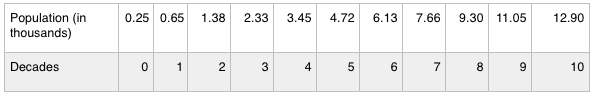Assuming that this species of rabbits breeds at the same rate, how many rabbits would there be in 20 years if the scientists had introduced 500 rabbits into the environment instead of 250?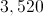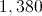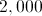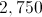Explanation:

This question is a little tricky. We can see that the relationship between the variables is not linear, so doubling the original number of rabbits does not necessarily double the number after 20 years.

If there are 500 rabbits to start, then we can look at the table between the starting year and the first decade to see where this population may exist in our given data. At some point during the first decade, as the population increased from 250 to 650 rabbits, it passed the population of 500. Since we know that the 500 population existed somewhere between year 0 and year 10, we can add 20 years to estimate the population after 20 years, if it started with 500 rabbits.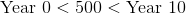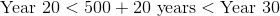Substitute the populations that we know.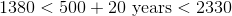Our answer will be somewhere between 1380 and 2330, likely slightly closer to the higher value. Looking at our answer choices, we can choose 2,000.

### Example Question #42 : How To Find Synthesis Of Data In Biology

There is a certain species of rabbit that is known to reproduce quickly. 250 of these rabbits were introduced into a new environment, and their population was measured in ten year intervals.Based on the table above, how many rabbits should there be in 11 decades?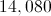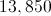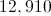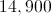Explanation:

We can look at the last few decades of the table to estimate how the population will increase beyond the scope of the experiment.

Increase from decade 7 to 8: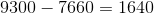Increase from decade 8 to 9: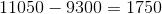Increase from decade 9 to 10: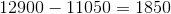Based on the trend, the next decade should show an increase of around 1,950, or a little more.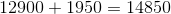Based on this estimate, we can choose the answer to be 14,900.

### Example Question #41 : How To Find Synthesis Of Data In Biology

There is a certain species of rabbit that is known to reproduce quickly. 250 of these rabbits were introduced into a new environment, and their population was measured in ten year intervals.Assuming the same rate of change, how many rabbits would there be after one decade in the scientists had started with 650 rabbits instead of 250?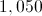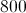Explanation:

After one decade in the original experiment, there were 650 rabbits. One decade later (decade two in the experiment), there were 1,380 rabbits. If they had started with 650 rabbits, they would have had 1,380 rabbits after one decade.

### Example Question #42 : How To Find Synthesis Of Data In Biology

There is a certain species of rabbit that is known to reproduce quickly. 250 of these rabbits were introduced into a new environment, and their population was measured in ten year intervals.All rabbits in the population are either brown or white. After each decade, it is discovered that there is a constant ratio of two brown rabbits for every three white rabbits. Which of the following conclusions can be drawn?

White rabbits and brown rabbits reproduce with the same frequency

White rabbits reproduce more frequently than brown rabbits

No conclusion can be drawn

Brown rabbits are more likely to be eaten by predators

White rabbits and brown rabbits reproduce with the same frequency

Explanation:

If the ratio of brown to white rabbits is two to three, that means that for every two brown rabbits, there are three white rabbits. This ratio is constant across the decades.

This means that after 10 years, of the 650 rabbits in the population, 260 are brown and 390 are white.

After 20 years, of the 1,380 rabbits in the population, 552 are brown and 828 are white.

We can compare the increases in each color: there are 292 new brown rabbits and 438 new white rabbits. So, there are definitely more white rabbits than brown rabbits, but this does not tell us the rate of population growth. To find the rate of increase we need to use this formula: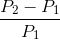This will give us the amount of change, relative to the starting amount.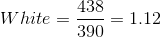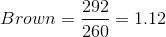Both populations increase by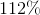, showing identical rates of increase. This is necessary in order to maintain a constant color ratio. If the white rabbits had a greater reproductive rate (or the brown rabbits a lower one), then the ratio would increase in favor of the white rabbits as they slowly overwhelmed the brown rabbit population.

### Example Question #42 : How To Find Synthesis Of Data In Biology

There is a certain species of rabbit that is known to reproduce quickly. 250 of these rabbits were introduced into a new environment, and their population was measured in ten year intervals.What is the relationship between population and decade?

Direct relationship

Indirect relationship

Exponential relationship

Exponential relationship

Explanation:

As the decade increases, the population also increases. This would indicate a direct relationship, except that the increase per decade is not constant. A direct relationship implies that the dependent variable can be graphed as a constant times the independent variable; this experiment cannot be graphed with a constant. Instead, we can see that the rate of population growth is increasing, suggesting an exponential relationship between population and decade.

### Example Question #47 : How To Find Synthesis Of Data In Biology

Chemical reactions involve two main components, reactants and products. The reactants, often referred to as substrates, interact with each other and rearrange in order to be converted into products. The speeds of these reactions are often defined by substrate concentration and the presence of enzymes. Enzymes are referred to as catalysts. Peroxidase is traditionally derived from turnips; however, it is commonly found in many plant and animal cells. This enzyme helps plant cells by removing hydrogen peroxide from cells in the form of tetraguaiacol.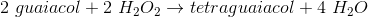Study 1

A scientist wants to observe the production of tetraguaiacol by observing a reaction between hydrogen peroxide and guaiacol. The product of this reaction is orange-brown in color. The scientist measures the intensity of color in each sample using a spectrophotometer. In the control experiment, the scientist mixed the substrates together and measured the reaction rate. In the test experiment, a peroxidase enzyme was added to a new set of substrates and rate of reaction was measured. The results of these reactions are plotted in Figure 1.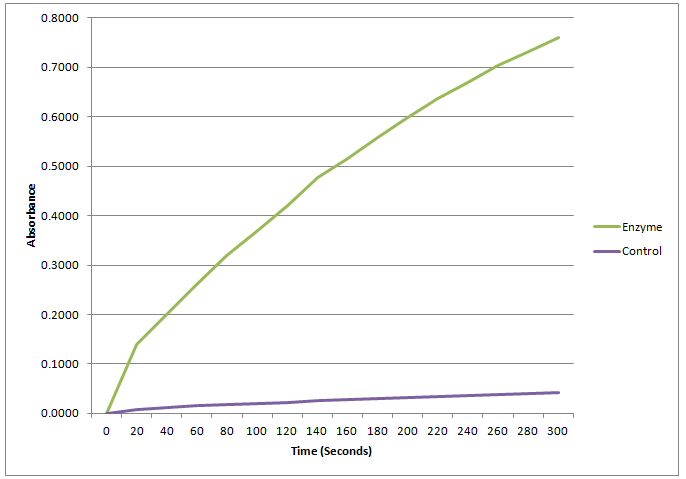Figure 1

Study 2

A research team decides to study the effects of the peroxidase facilitated reaction in the presence of heat. Reaction rates are known to speed up when heat is applied; however, at a certain point enzymes, such as peroxidase, denature and the reaction slows. The scientists perform a control trial at room temperature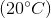and test trials at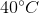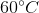, and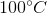. The results are plotted in Figure 2.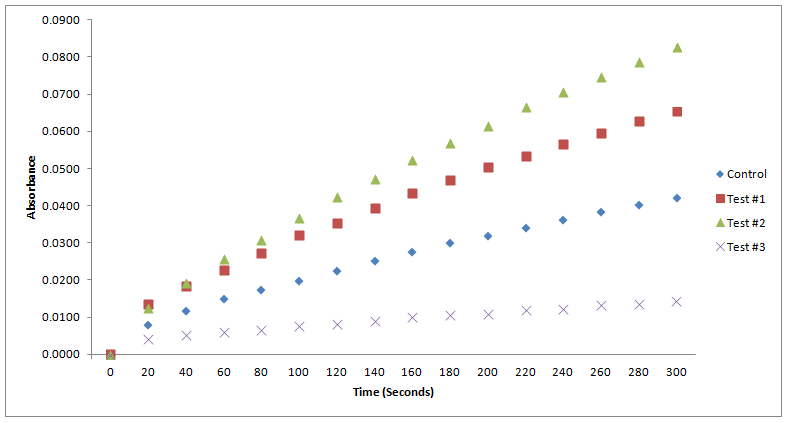Figure 2

According to the passage, how may moles of guaiacol would have to react in excess of hydrogen peroxide to produce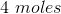of tetraguaiacol?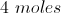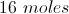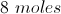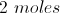Explanation:

The question states that the reaction occurs in excess of hydrogen peroxide. This means that there is more than enough hydrogen peroxide present during the reaction to create theof tetraguaiacol. Using the chemical formula in the passage we can easily calculate how many moles of guaiacol are needed to complete this reaction.The ratio of guaiacol to tetraguaiacol is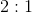.

Therefore, in order to produceof tetraguaiacol one would need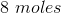of guaiacol.

### Example Question #48 : How To Find Synthesis Of Data In Biology

Chemical reactions involve two main components, reactants and products. The reactants, often referred to as substrates, interact with each other and rearrange in order to be converted into products. The speeds of these reactions are often defined by substrate concentration and the presence of enzymes. Enzymes are referred to as catalysts. Peroxidase is traditionally derived from turnips; however, it is commonly found in many plant and animal cells. This enzyme helps plant cells by removing hydrogen peroxide from cells in the form of tetraguaiacol.Study 1

A scientist wants to observe the production of tetraguaiacol by observing a reaction between hydrogen peroxide and guaiacol. The product of this reaction is orange-brown in color. The scientist measures the intensity of color in each sample using a spectrophotometer. In the control experiment, the scientist mixed the substrates together and measured the reaction rate. In the test experiment, a peroxidase enzyme was added to a new set of substrates and rate of reaction was measured. The results of these reactions are plotted in Figure 1.Figure 1

Study 2

A research team decides to study the effects of the peroxidase facilitated reaction in the presence of heat. Reaction rates are known to speed up when heat is applied; however, at a certain point enzymes, such as peroxidase, denature and the reaction slows. The scientists perform a control trial at room temperatureand test trials at,, and. The results are plotted in Figure 2.Figure 2

Did peroxidase increase the amount of tetraguaicol produced in Figure1?

Peroxidase had no effect on the reaction.

Peroxidase decreased the amount of tetraguaiacol produced in the reaction.

Peroxidase greatly increased the amount of tetraguaicol produced in the reaction.

Peroxidase slightly increased the amount of tetraguaiacol produced in the reaction.

Peroxidase greatly increased the amount of tetraguaicol produced in the reaction.

Explanation:

Peroxidase greatly increased the amount of tetraguaicol produced in the reaction.

The test group in Figure 1 contained peroxidase. When compared to the control, it is obvious that it greatly increased the amount of tetraguaiacol produced over the 300-second period. Tetraguaiacol production was greatly facilitated by the enzyme in the test. The other answers are incorrect because peroxidase's effect was not subtle, immeasurable, or detrimental to the production of tetraguaicol.

### Example Question #49 : How To Find Synthesis Of Data In Biology

Chemical reactions involve two main components, reactants and products. The reactants, often referred to as substrates, interact with each other and rearrange in order to be converted into products. The speeds of these reactions are often defined by substrate concentration and the presence of enzymes. Enzymes are referred to as catalysts. Peroxidase is traditionally derived from turnips; however, it is commonly found in many plant and animal cells. This enzyme helps plant cells by removing hydrogen peroxide from cells in the form of tetraguaiacol.Study 1

A scientist wants to observe the production of tetraguaiacol by observing a reaction between hydrogen peroxide and guaiacol. The product of this reaction is orange-brown in color. The scientist measures the intensity of color in each sample using a spectrophotometer. In the control experiment, the scientist mixed the substrates together and measured the reaction rate. In the test experiment, a peroxidase enzyme was added to a new set of substrates and rate of reaction was measured. The results of these reactions are plotted in Figure 1.Figure 1

Study 2

A research team decides to study the effects of the peroxidase facilitated reaction in the presence of heat. Reaction rates are known to speed up when heat is applied; however, at a certain point enzymes, such as peroxidase, denature and the reaction slows. The scientists perform a control trial at room temperatureand test trials at, and. The results are plotted in Figure 2.Figure 2

According to the passage, guiacol rids cells of hydrogen peroxide by producing what two products?

Four guaiacols, water, and hydrogen gas

Tetraguaiacol and water

Hydrogen peroxide and water

Tetraguaicol and hydrogen gas

Tetraguaiacol and water

Explanation:

Tetraguaiacol and water

The passage contains the chemical formula of the reaction.The products of the reaction are located on the right side of the arrow. This reaction produces 1 mole of tetraguaiacol and four moles of water. Water's chemical formula is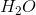because it contains two hydrogen atoms and one oxygen atom.

### Example Question #331 : Biology

Chemical reactions involve two main components, reactants and products. The reactants, often referred to as substrates, interact with each other and rearrange in order to be converted into products. The speeds of these reactions are often defined by substrate concentration and the presence of enzymes. Enzymes are referred to as catalysts. Peroxidase is traditionally derived from turnips; however, it is commonly found in many plant and animal cells. This enzyme helps plant cells by removing hydrogen peroxide from cells in the form of tetraguaiacol.Study 1

A scientist wants to observe the production of tetraguaiacol by observing a reaction between hydrogen peroxide and guaiacol. The product of this reaction is orange-brown in color. The scientist measures the intensity of color in each sample using a spectrophotometer. In the control experiment, the scientist mixed the substrates together and measured the reaction rate. In the test experiment, a peroxidase enzyme was added to a new set of substrates and rate of reaction was measured. The results of these reactions are plotted in Figure 1.Figure 1

Study 2

A research team decides to study the effects of the peroxidase facilitated reaction in the presence of heat. Reaction rates are known to speed up when heat is applied; however, at a certain point enzymes, such as peroxidase, denature and the reaction slows. The scientists perform a control trial at room temperatureand test trials at, and. The results are plotted in Figure 2.Figure 2

In Figure 2 of Study 2, the scientist observed the enzyme facilitated reaction in the presence of heat. What could explain the results found in Test 1 and Test 2?

The test reactions occurred faster than the control due to increased enzyme levels.

The test reactions occurred faster than the control due to increased heat application.

The test reactions did not differ from the control trial.

One of the test reactions occured faster and one occured slower than the control trial.